#### Fields

Polynomials are usually defined over a field, which is another object from algebra. A field is similar to a group, but it has more operations and axioms. The definition is given below, and while reading it, keep in mind several familiar examples of fields: the rationals,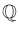; the reals,; and the complex plane,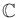. You may verify that these fields satisfy the following six axioms.

A field is a set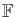that has two binary operations,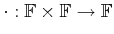(called multiplication) and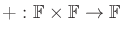(called addition), for which the following axioms are satisfied:

1. (Associativity) For all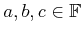,and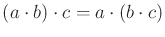.
2. (Commutativity) For all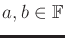,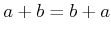and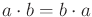.
3. (Distributivity) For all,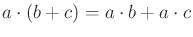.
4. (Identities) There exist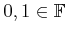, such that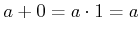for all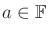.
5. (Additive Inverses) For every, there exists some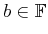such that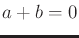.
6. (Multiplicative Inverses) For every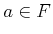, except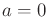, there exists some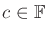such that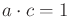.
Compare these axioms to the group definition from Section 4.2.1. Note that a field can be considered as two different kinds of groups, one with respect to multiplication and the other with respect to addition. Fields additionally require commutativity; hence, we cannot, for example, build a field from quaternions. The distributivity axiom appears because there is now an interaction between two different operations, which was not possible with groups.

Steven M LaValle 2020-08-14